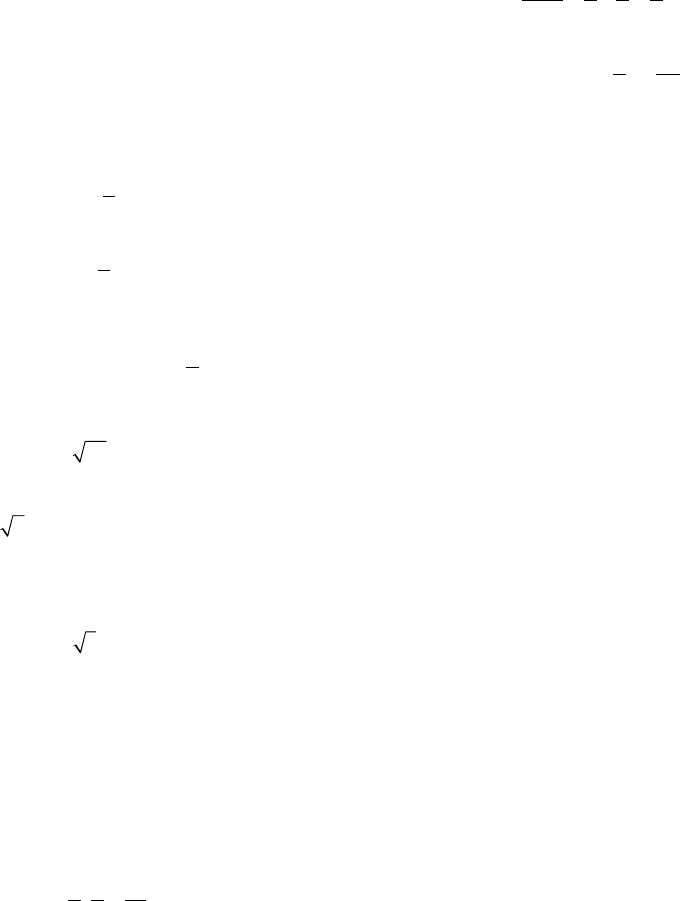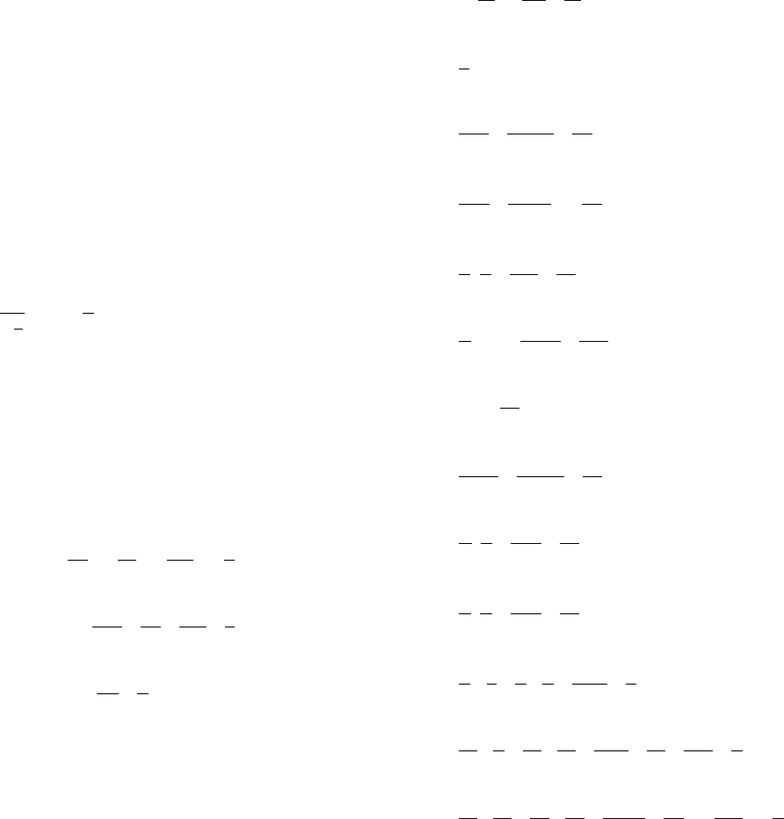# MATA 32&33 Solution Manual.pdf

243 views719 pages
School
UTSC
Department
Mathematics
Course
MATA32H3
Chapter 0 1
Chapter 1 35
Chapter 2 54
Chapter 3 89
Chapter 4 132
Chapter 5 160
Chapter 6 177
Chapter 7 231
Chapter 8 295
Chapter 9 333
Chapter 10 357
Chapter 11 378
Chapter 12 423
Chapter 13 469
Chapter 14 539
Chapter 15 614
Chapter 16 658
Chapter 17 670
Unlock document

This preview shows pages 1-3 of the document.
Unlock all 719 pages and 3 million more documents.1
Chapter 0
Problems 0.1
1. True; –13 is a negative integer.
2. True, because 2 and 7 are integers and 7 0.
3. False, because the natural numbers are 1, 2, 3,
and so on.
4. False, because 0
0.
1
=
5. True, because 5
5.
1
=
6. False, since a rational number cannot have
denominator of zero. In fact, 7
0 is not a number
at all because we cannot divide by 0.
7. False, because 25 5,= which is a positive
integer.
8. True; 2 is an irrational real number.
9. False; we cannot divide by 0.
10. False, because the natural numbers are 1, 2, 3,
and so on, and 3 lies between 1 and 2.
11. True
12. False, since the integer 0 is neither positive nor
negative.
Problems 0.2
1. False, because 0 does not have a reciprocal.
2. True, because
73 21 1.
37 21
⋅= =
3. False; the negative of 7 is 7 because
7 + (7) = 0.
4. False; 2(3 · 4) = 2(12) = 24, but
(2 · 3)(2 · 4) = 6 · 8 = 48.
5. False; x + y = y + (–x) = yx.
6. True; (x + 2)(4) = (x)(4) + (2)(4) = 4x + 8.
7. True; 22
1.
2222
xxx+
=
+=+
8. True, because .
bab
acc
⎛⎞
=
⎜⎟
⎝⎠
9. False; the left side is 5xy, but the right side is
2
5.
x
y
10. True; by the associative and commutative
properties, x(4y) = (x 4)y = (4 x)y = 4xy.
11. distributive
12. commutative
13. associative
14. definition of division
15. commutative and distributive
16. associative
17. definition of subtraction
18. commutative
19. distributive
20. distributive
21. 2x(y 7) = (2x)y (2x)7 = 2xy (7)(2x)
= 2xy (7 · 2)x = 2xy 14x
22. (a b) + c = [a + (b)] + c = a + (b + c)
= a + [c + (b)] = a + (c b)
23. (x + y)(2) = 2(x + y) = 2x + 2y
24. 2[27 + (x + y)] = 2[27 + (y + x)] = 2[(27 + y) + x]
= 2[(y + 27) + x]
25. x[(2y + 1) + 3] = x[2y + (1 + 3)] = x[2y + 4]
= x(2y) + x(4) = (x · 2)y + 4x = (2x)y + 4x
= 2xy + 4x
26. (1 + a)(b + c) = 1(b + c) + a(b + c)
= 1(b) + 1(c) + a(b) + a(c) = b + c + ab + ac
Unlock document

This preview shows pages 1-3 of the document.
Unlock all 719 pages and 3 million more documents.Chapter 0: Review of Algebra ISM: Introductory Mathematical Analysis
2
27. x(y z + w) = x[(y z) + w] = x(y z) + x(w)
= x[y + (z)] + xw = x(y) + x(z) + xw
= xy xz + xw
28. –2 + (–4) = –6
29. –6 + 2 = –4
30. 6 + (–4) = 2
31. 7 – 2 = 5
32. 7 – (–4) = 7 + 4 = 11
33. 5 (13) = 5 + 13 = 8
34. a (b) = a + b
35. (–2)(9) = –(2 · 9) = –18
36. 7(–9) = –(7 · 9) = –63
37. (–2)(–12) = 2(12) = 24
38. 19(1) = (1)19 = (1 · 19) = 19
39. 1
9
19
19
1
⎛⎞
=− − =
⎜⎟
⎝⎠
40. –(–6 + x) = –(–6) – x = 6 – x
41. –7(x) = –(7x) = –7x
42. –12(xy) = (–12)x – (–12)(y) = –12x + 12y
(or 12y – 12x)
43. –[–6 + (–y)] = –(–6) – (–y) = 6 + y
44. 33131
315 15 15 5 3 5
−⋅
−÷ = = = =
45. 99911
9(27) 27 27 9 3 3
−⋅
−÷− = = = =
−⋅
46. ()() aa
abbb
−÷= =
47. 2(–6 + 2) = 2(–4) = –8
48. 3[–2(3) + 6(2)] = 3[–6 + 12] = 3 = 18
49. (–2)(–4)(–1) = 8(–1) = –8
50. (12)(12) = (12)(12) = 144
51. X(1) = X
52. 3(x – 4) = 3(x) – 3(4) = 3x – 12
53. 4(5 + x) = 4(5) + 4(x) = 20 + 4x
54. –(x – 2) = –x + 2
55. 0(–x) = 0
56. 1818
811 11 11
⎛⎞
=
=
⎜⎟
⎝⎠
57. 55
1
=
58. 14 2 7 2
21 3 7 3
x
xx
yyy
⋅⋅
==
⋅⋅
59. 33 3
2(2)2
x
xx
==
−−
60. 21 21 2
333
x
xx
⋅= =
61. (3 ) 3
(3 )
aabab
b
ccc
==
62. 7
(5 ) 7
5
aa
⎛⎞
⎜⎟
⎝⎠
63. aby a by by
ax a x x
−−
==
−−
64. 71 71 7
yx yx xy
⋅= =
65. 25 25 10
x
yxyxy
⋅= =
66. 1132325
2366 6 6
+
+
=+= =
67. 535 95914277
12 4 12 12 12 12 2 6 6
+⋅
+
=+= == =
68. 37 914914 5 51 1
10 15 30 30 30 30 5 6 6
−− ⋅
=−= ==− =
Unlock document

This preview shows pages 1-3 of the document.
Unlock all 719 pages and 3 million more documents.